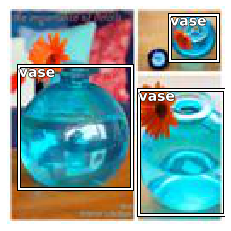Basic image opening/processing functionality
from nbdev.showdoc import *
from fastai2.data.external import *

#TODO: investigate


## Helpers

im = Image.open(TEST_IMAGE).resize((30,20))


#### n_px[source]

n_px(x:Image)

#### Image.n_px

Image.n_px (property)

Number of pixels in image

test_eq(im.n_px, 30*20)


#### shape[source]

shape(x:Image)

#### Image.shape

Image.shape (property)

Image (height,width) tuple (NB:opposite order of Image.size(), same order as numpy array and pytorch tensor)

test_eq(im.shape, (20,30))


#### aspect[source]

aspect(x:Image)

#### Image.aspect

Image.aspect (property)

Aspect ratio of the image, i.e. width/height

test_eq(im.aspect, 30/20)


#### Image.reshape[source]

Image.reshape(x:Image, h, w, resample=0)

resize x to (w,h)

test_eq(im.reshape(12,10).shape, (12,10))


#### Image.to_bytes_format[source]

Image.to_bytes_format(im:Image, format='png')

Convert to bytes, default to PNG format

#### Image.to_thumb[source]

Image.to_thumb(h, w=None)

Same as thumbnail, but uses a copy

test_eq(im.resize_max(max_px=20*30).shape, (20,30))
test_eq(im.resize_max(max_px=300).n_px, 294)
test_eq(im.resize_max(max_px=500, max_h=10, max_w=20).shape, (10,15))
test_eq(im.resize_max(max_h=14, max_w=15).shape, (10,15))
test_eq(im.resize_max(max_px=300, max_h=10, max_w=25).shape, (10,15))


#### Image.resize_max[source]

Image.resize_max(x:Image, resample=0, max_px=None, max_h=None, max_w=None)

resize x to max_px, or max_h, or max_w

## Basic types

This section regroups the basic types used in vision with the transform that create objects of those types.

# TODO: docs


#### load_image[source]

load_image(fn, mode=None, **kwargs)

Open and load a PIL.Image and convert to mode

#### image2tensor[source]

image2tensor(img)

Transform image to byte tensor in c*h*w dim order.

### classPILBase[source]

PILBase() :: Image

This class represents an image object. To create :py:class:~PIL.Image.Image objects, use the appropriate factory functions. There's hardly ever any reason to call the Image constructor directly.

• :py:func:~PIL.Image.open
• :py:func:~PIL.Image.new
• :py:func:~PIL.Image.frombytes

### classPILImage[source]

PILImage() :: PILBase

This class represents an image object. To create :py:class:~PIL.Image.Image objects, use the appropriate factory functions. There's hardly ever any reason to call the Image constructor directly.

• :py:func:~PIL.Image.open
• :py:func:~PIL.Image.new
• :py:func:~PIL.Image.frombytes

### classPILImageBW[source]

PILImageBW() :: PILImage

This class represents an image object. To create :py:class:~PIL.Image.Image objects, use the appropriate factory functions. There's hardly ever any reason to call the Image constructor directly.

• :py:func:~PIL.Image.open
• :py:func:~PIL.Image.new
• :py:func:~PIL.Image.frombytes
im = PILImage.create(TEST_IMAGE)
test_eq(type(im), PILImage)
test_eq(im.mode, 'RGB')
test_eq(str(im), 'PILImage mode=RGB size=1200x803')

im.resize((64,64))ax = im.show(figsize=(1,1))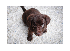test_fig_exists(ax)

timg = TensorImage(image2tensor(im))
tpil = PILImage.create(timg)

tpil.resize((64,64))### classPILMask[source]

PILMask() :: PILBase

This class represents an image object. To create :py:class:~PIL.Image.Image objects, use the appropriate factory functions. There's hardly ever any reason to call the Image constructor directly.

• :py:func:~PIL.Image.open
• :py:func:~PIL.Image.new
• :py:func:~PIL.Image.frombytes
im = PILMask.create(TEST_IMAGE)
test_eq(im.mode, 'L')


### Images

mnist = untar_data(URLs.MNIST_TINY)
fns = get_image_files(mnist)
mnist_fn = TEST_IMAGE_BW

timg = Transform(PILImageBW.create)
mnist_img = timg(mnist_fn)
test_eq(mnist_img.size, (28,28))
assert isinstance(mnist_img, PILImageBW)
mnist_img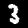### classAddMaskCodes[source]

AddMaskCodes(codes=None) :: Transform

Add the code metadata to a TensorMask

camvid = untar_data(URLs.CAMVID_TINY)
fns = get_image_files(camvid/'images')
cam_fn = fns

cam_img = PILImage.create(cam_fn)
test_eq(cam_img.size, (128,96))

_,axs = plt.subplots(1,3, figsize=(12,3))
cam_img.show(ctx=axs, title='image')
cam_img.show(ctx=axs, title='superimposed')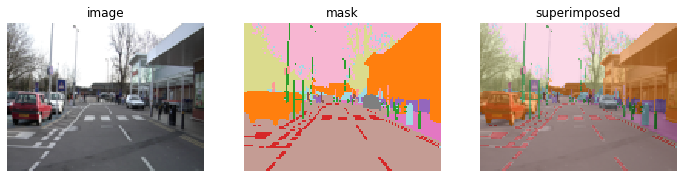### classTensorPoint[source]

TensorPoint(x, **kwargs) :: TensorBase

Basic type for points in an image

Points are expected to come as an array/tensor of shape (n,2) or as a list of lists with two elements. Unless you change the defaults in PointScaler (see later on), coordinates should go from 0 to width/height, with the first one being the column index (so from 0 to width) and the second one being the row index (so from 0 to height).

pnt_img = TensorImage(mnist_img.resize((28,35)))
pnts = np.array([[0,0], [0,35], [28,0], [28,35], [9, 17]])
tfm = Transform(TensorPoint.create)
tpnts = tfm(pnts)
test_eq(tpnts.shape, [5,2])
test_eq(tpnts.dtype, torch.float32)

ctx = pnt_img.show(figsize=(1,1), cmap='Greys')
tpnts.show(ctx=ctx);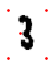### Bounding boxes

#### get_annotations[source]

get_annotations(fname, prefix=None)

Open a COCO style json in fname and returns the lists of filenames (with maybe prefix) and labelled bboxes.

### classTensorBBox[source]

TensorBBox(x, **kwargs) :: TensorPoint

Basic type for a tensor of bounding boxes in an image

Bounding boxes are expected to come as tuple with an array/tensor of shape (n,4) or as a list of lists with four elements and a list of corresponding labels. Unless you change the defaults in PointScaler (see later on), coordinates for each bounding box should go from 0 to width/height, with the following convention: x1, y1, x2, y2 where (x1,y1) is your top-left corner and (x2,y2) is your bottom-right corner.

### classLabeledBBox[source]

LabeledBBox(items=None, *rest, use_list=False, match=None) :: L

Basic type for a list of bounding boxes in an image

coco = untar_data(URLs.COCO_TINY)
images, lbl_bbox = get_annotations(coco/'train.json')
idx=2
coco_fn,bbox = coco/'train'/images[idx],lbl_bbox[idx]
coco_img = timg(coco_fn)

tbbox = LabeledBBox(TensorBBox(bbox), bbox)
ctx = coco_img.show(figsize=(3,3), cmap='Greys')
tbbox.show(ctx=ctx);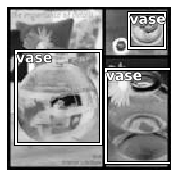## Basic Transforms

Unless specifically mentioned, all the following transforms can be used as single-item transforms (in one of the list in the tfms you pass to a TfmdDS or a Datasource) or tuple transforms (in the tuple_tfms you pass to a TfmdDS or a Datasource). The safest way that will work across applications is to always use them as tuple_tfms. For instance, if you have points or bounding boxes as targets and use Resize as a single-item transform, when you get to PointScaler (which is a tuple transform) you won't have the correct size of the image to properly scale your points.

#### encodes[source]

encodes(x:TensorBBox)

#### encodes[source]

encodes(x:TensorBBox)

Any data augmentation transform that runs on PIL Images must be run before this transform.

tfm = ToTensor()
print(tfm)
print(type(mnist_img))
print(type(tfm(mnist_img)))

ToTensor: (PILMask,object) -> encodes
(PILBase,object) -> encodes
<class '__main__.PILImageBW'>
<class 'fastai2.torch_core.TensorImageBW'>

tfm = ToTensor()
test_eq(tfm(mnist_img).shape, (1,28,28))
test_eq(type(tfm(mnist_img)), TensorImageBW)


Let's confirm we can pipeline this with PILImage.create.

pipe_img = Pipeline([PILImageBW.create, ToTensor()])
img = pipe_img(mnist_fn)
test_eq(type(img), TensorImageBW)
pipe_img.show(img, figsize=(1,1));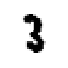def _cam_lbl(x): return mask_fn
cam_tds = Datasets([cam_fn], [[PILImage.create, ToTensor()], [_cam_lbl, PILMask.create, ToTensor()]])
show_at(cam_tds, 0);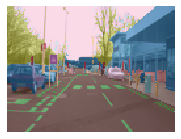To work with data augmentation, and in particular the grid_sample method, points need to be represented with coordinates going from -1 to 1 (-1 being top or left, 1 bottom or right), which will be done unless you pass do_scale=False. We also need to make sure they are following our convention of points being x,y coordinates, so pass along y_first=True if you have your data in an y,x format to add a flip.

### classPointScaler[source]

PointScaler(do_scale=True, y_first=False) :: Transform

Scale a tensor representing points

To work with data augmentation, and in particular the grid_sample method, points need to be represented with coordinates going from -1 to 1 (-1 being top or left, 1 bottom or right), which will be done unless you pass do_scale=False. We also need to make sure they are following our convention of points being x,y coordinates, so pass along y_first=True if you have your data in an y,x format to add a flip.

def _pnt_lbl(x): return TensorPoint.create(pnts)
def _pnt_open(fn): return PILImage(PILImage.create(fn).resize((28,35)))
pnt_tds = Datasets([mnist_fn], [_pnt_open, [_pnt_lbl]])
pnt_tdl = TfmdDL(pnt_tds, bs=1, after_item=[PointScaler(), ToTensor()])

test_eq(pnt_tdl.after_item.c, 10)

x,y = pnt_tdl.one_batch()
#Scaling and flipping properly done
#NB: we added a point earlier at (9,17); formula below scales to (-1,1) coords
test_close(y, tensor([[-1., -1.], [-1.,  1.], [1.,  -1.], [1., 1.], [9/14-1, 17/17.5-1]]))
a,b = pnt_tdl.decode_batch((x,y))
test_eq(b, tensor(pnts).float())
#Check types
test_eq(type(x), TensorImage)
test_eq(type(y), TensorPoint)
test_eq(type(a), TensorImage)
test_eq(type(b), TensorPoint)
test_eq(b.get_meta('img_size'), (28,35)) #Automatically picked the size of the input

pnt_tdl.show_batch(figsize=(2,2), cmap='Greys');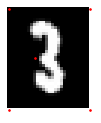### classBBoxLabeler[source]

BBoxLabeler(enc=None, dec=None, split_idx=None, order=None) :: Transform

Delegates (__call__,decode,setup) to (encodes,decodes,setups) if split_idx matches

#### decodes[source]

decodes(x:TensorBBox)

#### encodes[source]

encodes(x:TensorBBox)

#### decodes[source]

decodes(x:TensorBBox)

def _coco_bb(x):  return TensorBBox.create(bbox)
def _coco_lbl(x): return bbox

coco_tds = Datasets([coco_fn], [PILImage.create, [_coco_bb], [_coco_lbl, MultiCategorize(add_na=True)]], n_inp=1)
coco_tdl = TfmdDL(coco_tds, bs=1, after_item=[BBoxLabeler(), PointScaler(), ToTensor()])

x,y,z = coco_tdl.one_batch()
test_close(y, -1+tensor(bbox)/64)
test_eq(z, tensor([1,1,1]))
a,b,c = coco_tdl.decode_batch((x,y,z))
test_close(b, tensor(bbox).float())
test_eq(c.bbox, b)
test_eq(c.lbl, bbox)

#Check types
test_eq(type(x), TensorImage)
test_eq(type(y), TensorBBox)
test_eq(type(z), TensorMultiCategory)
test_eq(type(a), TensorImage)
test_eq(type(b), TensorBBox)
test_eq(type(c), LabeledBBox)
test_eq(y.get_meta('img_size'), (128,128))

coco_tdl.show_batch();